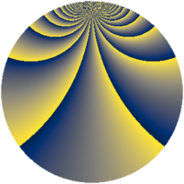# Properties

 Label 729.2.gLevel $729$ Weight $2$ Character orbit 729.g Rep. character $\chi_{729}(28,\cdot)$ Character field $\Q(\zeta_{27})$ Dimension $576$ Newform subspaces $4$ Sturm bound $162$ Trace bound $20$

# Related objects

## Defining parameters

 Level: $$N$$ $$=$$ $$729 = 3^{6}$$ Weight: $$k$$ $$=$$ $$2$$ Character orbit: $$[\chi]$$ $$=$$ 729.g (of order $$27$$ and degree $$18$$) Character conductor: $$\operatorname{cond}(\chi)$$ $$=$$ $$81$$ Character field: $$\Q(\zeta_{27})$$ Newform subspaces: $$4$$ Sturm bound: $$162$$ Trace bound: $$20$$ Distinguishing $$T_p$$: $$2$$

## Dimensions

The following table gives the dimensions of various subspaces of $$M_{2}(729, [\chi])$$.

Total New Old
Modular forms 1620 720 900
Cusp forms 1296 576 720
Eisenstein series 324 144 180

## Trace form

 $$576 q + 36 q^{4} + 36 q^{7} + O(q^{10})$$ $$576 q + 36 q^{4} + 36 q^{7} - 72 q^{10} + 36 q^{13} + 36 q^{16} - 72 q^{19} + 36 q^{22} + 36 q^{25} - 36 q^{28} + 36 q^{31} + 36 q^{34} - 72 q^{37} + 36 q^{40} + 36 q^{43} - 72 q^{46} + 36 q^{49} - 36 q^{55} + 36 q^{58} + 36 q^{61} - 72 q^{64} - 18 q^{67} - 72 q^{70} - 72 q^{73} - 126 q^{76} - 72 q^{79} - 144 q^{82} - 72 q^{85} - 126 q^{88} - 72 q^{91} - 72 q^{94} - 18 q^{97} + O(q^{100})$$

## Decomposition of $$S_{2}^{\mathrm{new}}(729, [\chi])$$ into newform subspaces

Label Dim $A$ Field CM Traces $q$-expansion
$a_{2}$ $a_{3}$ $a_{5}$ $a_{7}$
729.2.g.a $144$ $5.821$ None $$-9$$ $$0$$ $$-9$$ $$9$$
729.2.g.b $144$ $5.821$ None $$-9$$ $$0$$ $$-9$$ $$9$$
729.2.g.c $144$ $5.821$ None $$9$$ $$0$$ $$9$$ $$9$$
729.2.g.d $144$ $5.821$ None $$9$$ $$0$$ $$9$$ $$9$$

## Decomposition of $$S_{2}^{\mathrm{old}}(729, [\chi])$$ into lower level spaces

$$S_{2}^{\mathrm{old}}(729, [\chi]) \cong$$ $$S_{2}^{\mathrm{new}}(81, [\chi])$$$$^{\oplus 3}$$$$\oplus$$$$S_{2}^{\mathrm{new}}(243, [\chi])$$$$^{\oplus 2}$$